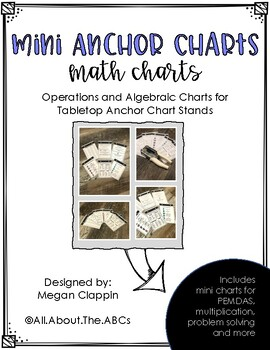# Math Mini Tabletop Anchor Charts: CCSS O.A.Subject
Resource Type
File Type

PDF

(54 MB|9 pages)
Product Rating
4.0
(1 Rating)
Standards
• Product Description
• StandardsNEW

This set of seven mini charts is designed to accompany your teaching with the CCSS OA Standards: Operations and Algebraic Expressions. Although useable for many elementary grade levels, these charts are primarily designed to be used with third through fifth graders. This is a set of mini anchor charts only. You will need IKEA Tolsby frames or a similar standing frame and my accompanying Mini Tabletop Anchor Chart Kit to complete the creation of these mini anchor charts. This download is a pdf format.

Generate two numerical patterns using two given rules. Identify apparent relationships between corresponding terms. Form ordered pairs consisting of corresponding terms from the two patterns, and graph the ordered pairs on a coordinate plane. For example, given the rule “Add 3” and the starting number 0, and given the rule “Add 6” and the starting number 0, generate terms in the resulting sequences, and observe that the terms in one sequence are twice the corresponding terms in the other sequence. Explain informally why this is so.
Write simple expressions that record calculations with numbers, and interpret numerical expressions without evaluating them. For example, express the calculation “add 8 and 7, then multiply by 2” as 2 × (8 + 7). Recognize that 3 × (18932 + 921) is three times as large as 18932 + 921, without having to calculate the indicated sum or product.
Use parentheses, brackets, or braces in numerical expressions, and evaluate expressions with these symbols.
Find all factor pairs for a whole number in the range 1-100. Recognize that a whole number is a multiple of each of its factors. Determine whether a given whole number in the range 1-100 is a multiple of a given one-digit number. Determine whether a given whole number in the range 1-100 is prime or composite.
Solve multistep word problems posed with whole numbers and having whole-number answers using the four operations, including problems in which remainders must be interpreted. Represent these problems using equations with a letter standing for the unknown quantity. Assess the reasonableness of answers using mental computation and estimation strategies including rounding.
Total Pages
9 pages
N/A
Teaching Duration
N/A
Report this Resource to TpT
Reported resources will be reviewed by our team. Report this resource to let us know if this resource violates TpT’s content guidelines.